# 事件触发驱动的非线性系统有限时间状态估计器设计*

(1. 上海工程技术大学 电子电气工程学院, 上海 201620;2. 上海立信会计金融学院 统计与数学学院, 上海 201620;3. 东华大学 信息科学与技术学院, 上海 201620)

## 1 问 题 描 述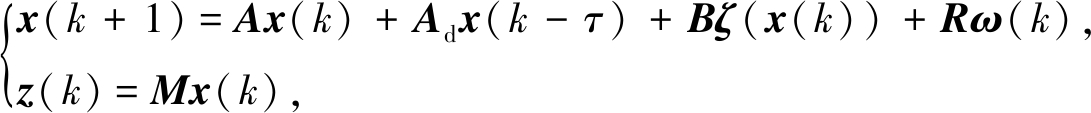(1)

y(k)=Cx(k)+Lv(k),

(2)

Ξk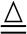y(k)-y(kp)‖2-θ

(3)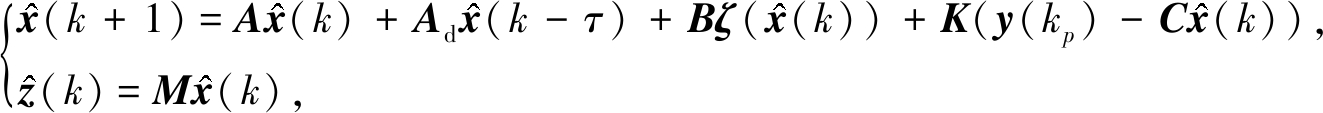(4)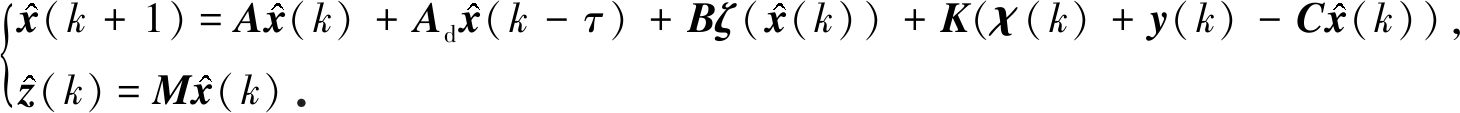(5)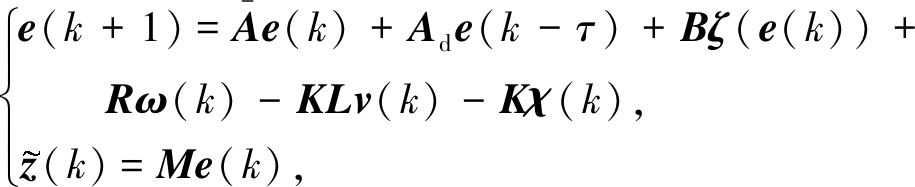(6)

|f(a)-f(b)|≤m(a-b),

(7)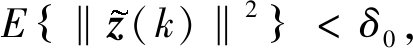(8)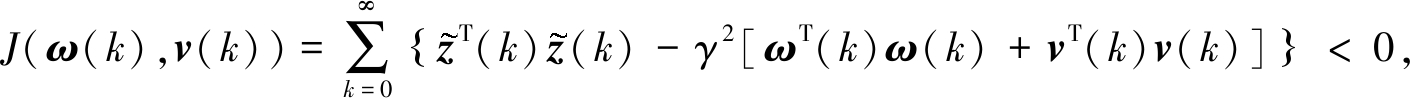(9)

## 2 主要定理及证明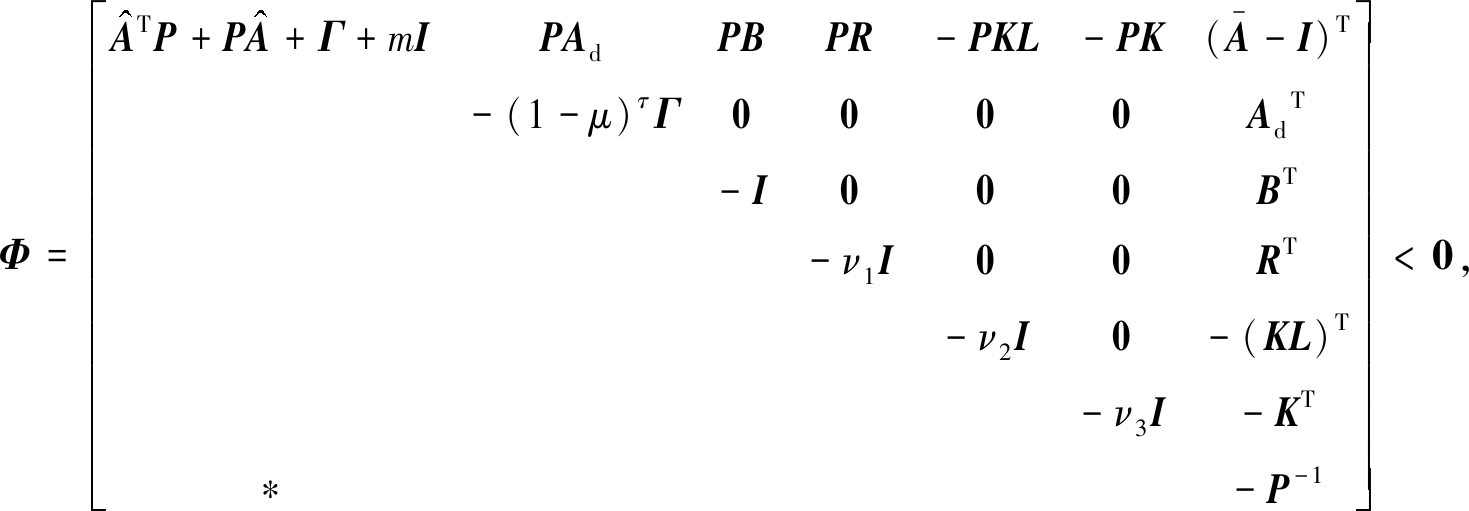(10)

δ / μ<δ0,

(11)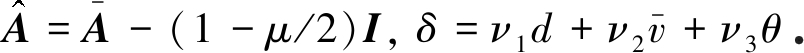(12)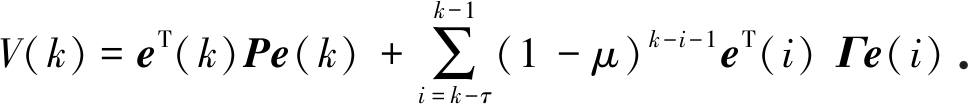(13)

V(k)两边取数学期望EV(k)}=E{V(k+1)-V(k)}

EV(k)+μV(k)}=

E{V(k+1)-(1-μ)V(k)}=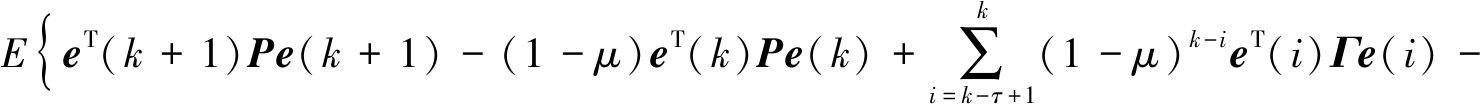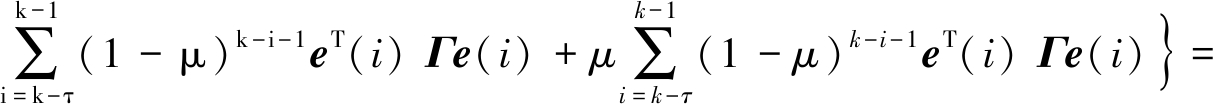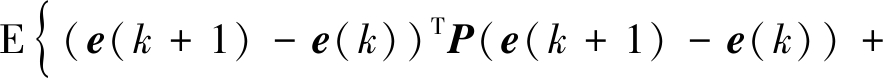2eT(k)P(e(k+1)-(1-μ/2)e(k))+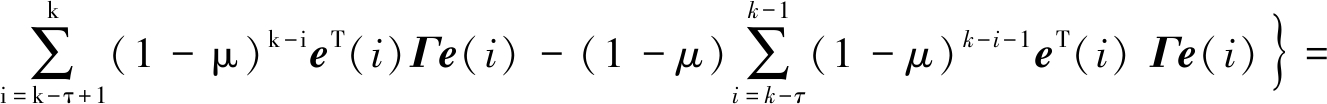E{(e(k+1)-e(k))TP(e(k+1)-e(k))+

2eT(k)P(e(k+1)-(1-μ/2)e(k))+

eT(k)Γe(k)-(1-μ)τeT(k-τ)Γe(k-τ)}

(14)

ζT(e(k))ζ(e(k))-meT(k)e(k)<0

(15)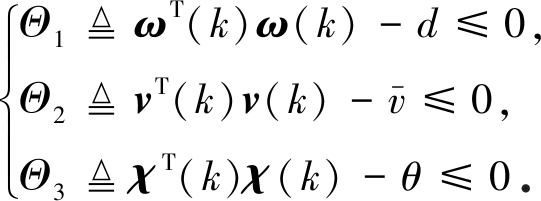(16)

EV(k)+μV(k)}≤

E{(e(k+1)-e(k))TP(e(k+1)-e(k))+

2eT(k)P(e(k+1)-(1-μ/2)e(k))+eT(k)Γe(k)-

(1-μ)τeT(k-τ)Γe(k-τ)-(ζT(e(k))ζ(e(k))-meT(k)e(k))-

ν1Θ1-ν2Θ2-ν3Θ3}=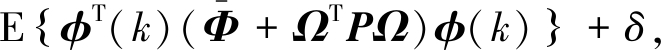(17)

φ(k)=[eT(k) eT(k-τ) ζT(e(k)) ω(k) v(k) χ(k)]T,

(18)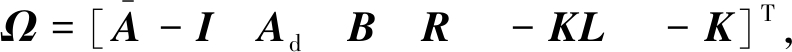(19)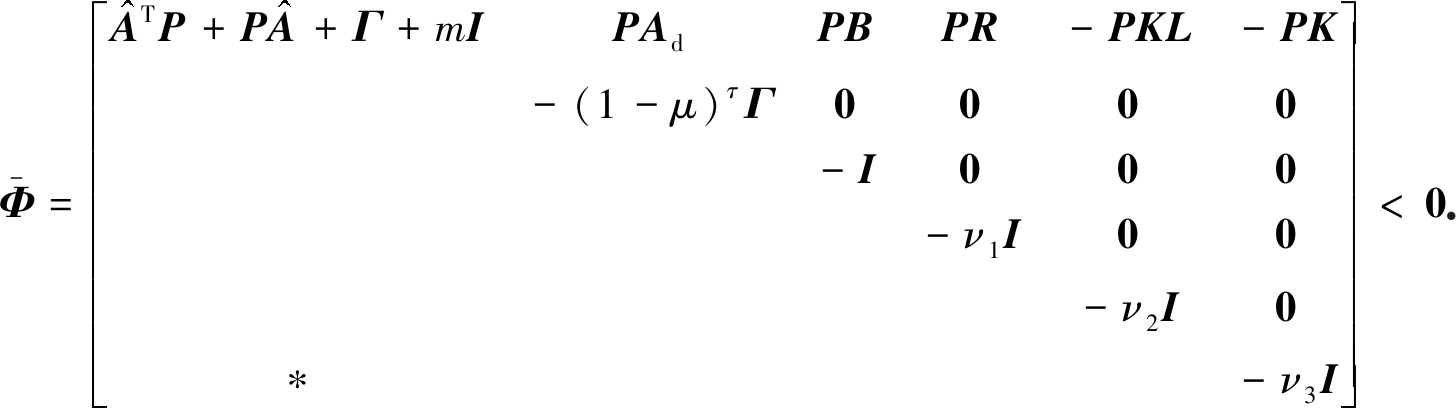(20)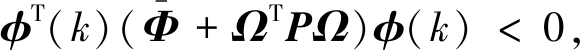(21)

EV(k)+μV(k)}<δ

(22)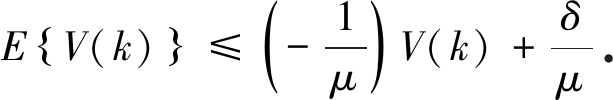(23)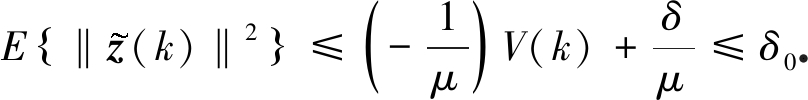(24)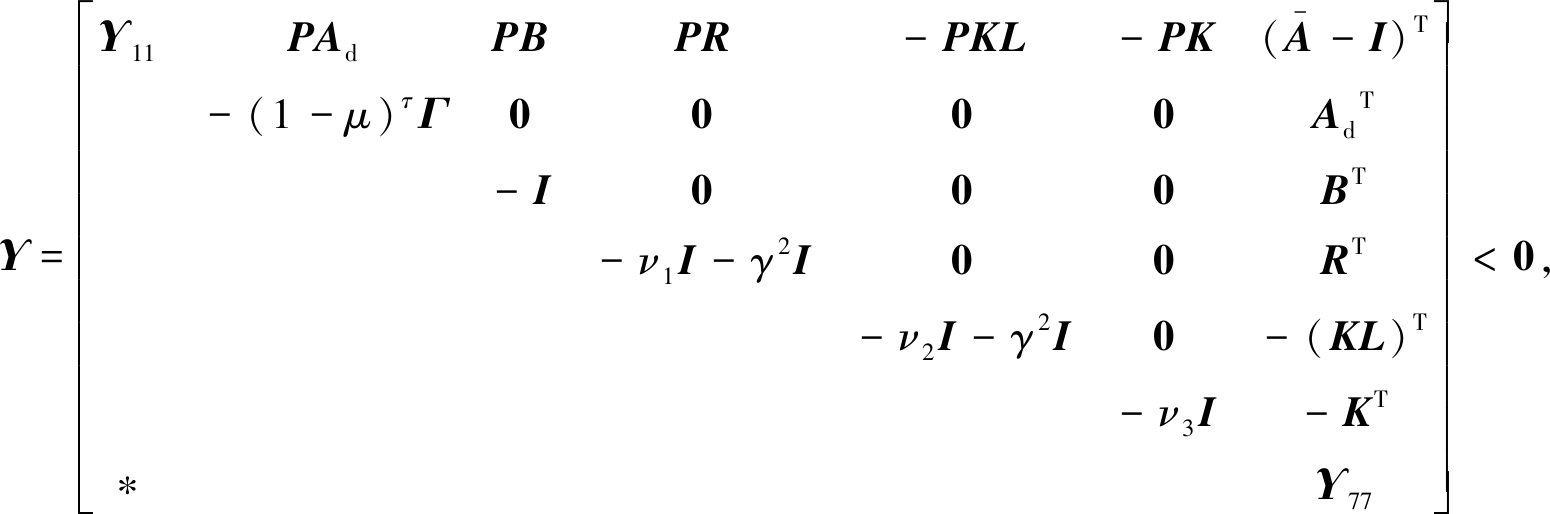(25)

δ/μ<δ0,

(26)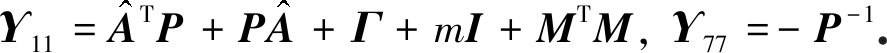(27)

J(ω(k),v(k))=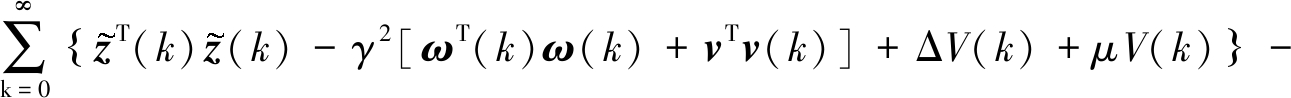{V(∞)-V(0)}-{μV(k)}≤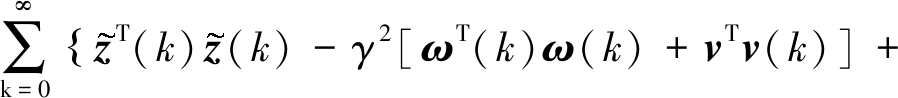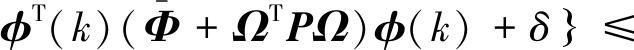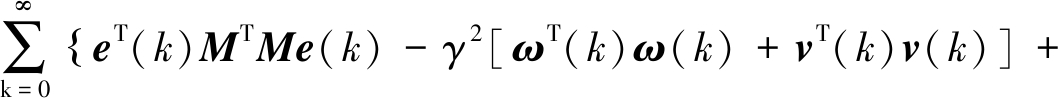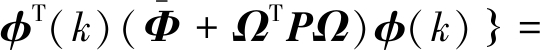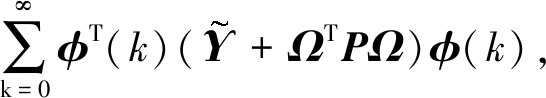(28)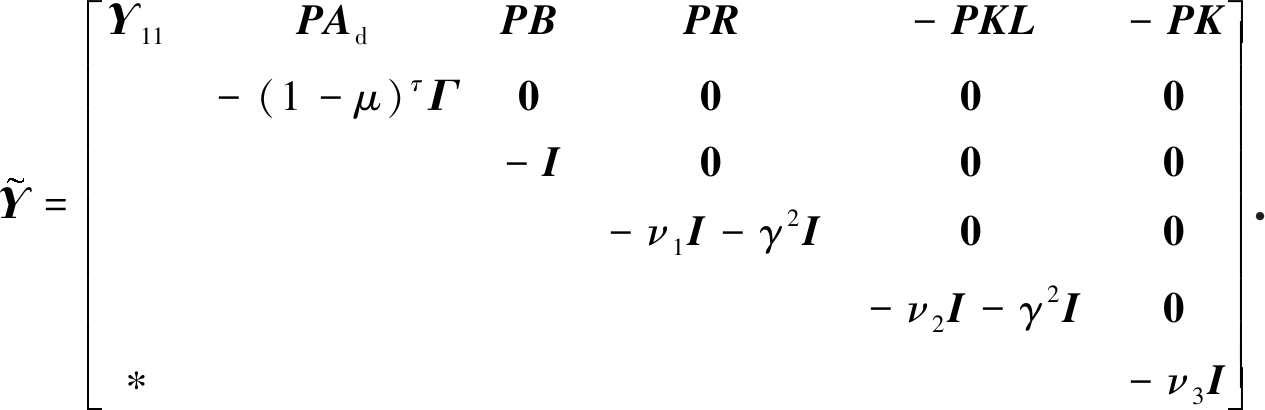(29)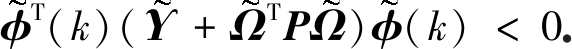(30)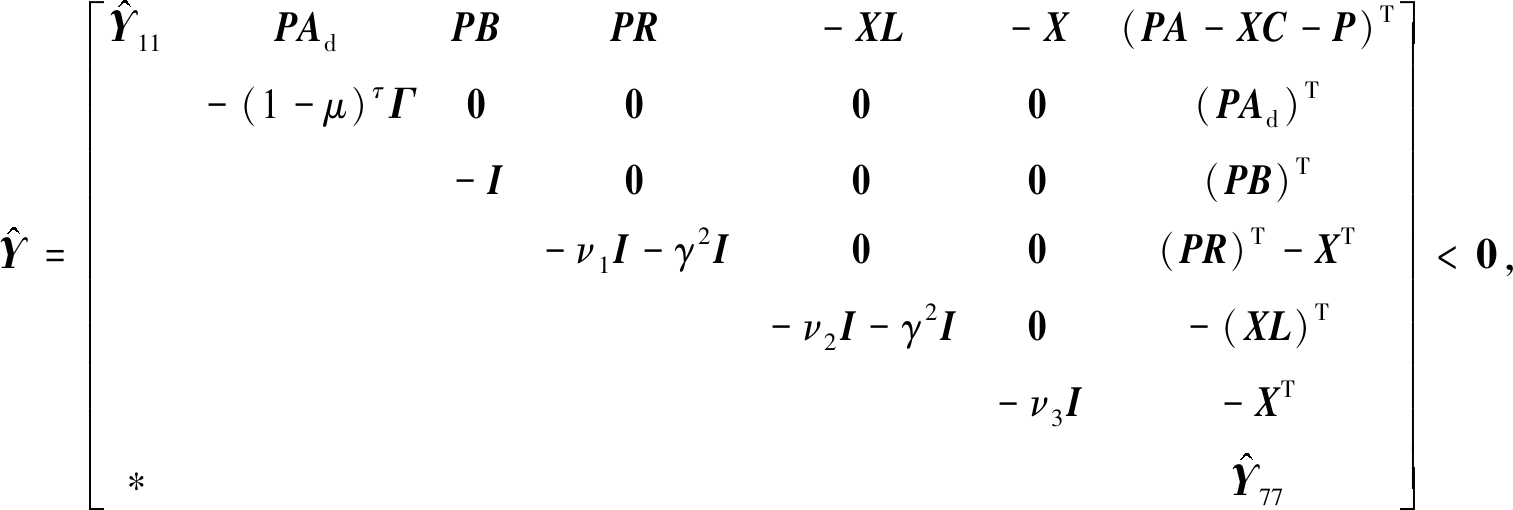(31)

δ/μ<δ0,

(32)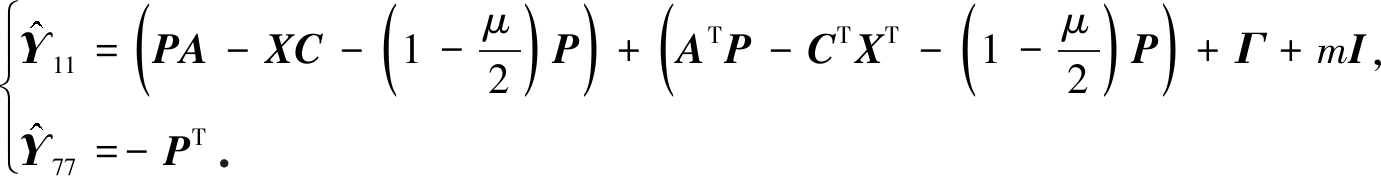(33)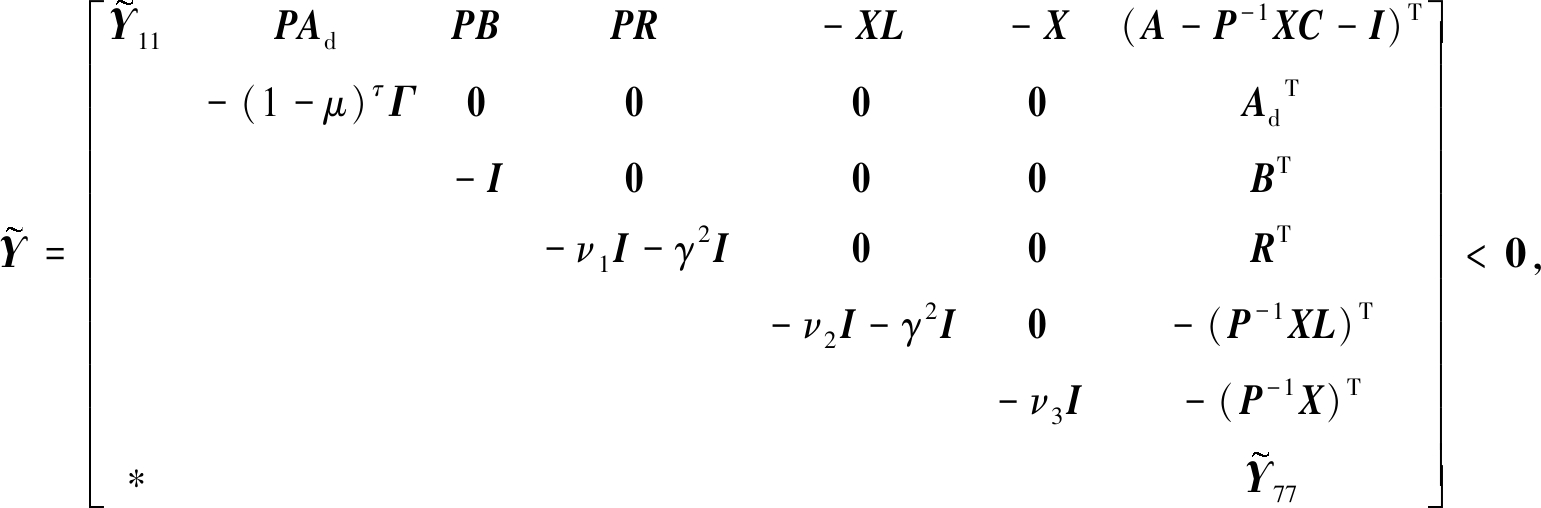(34)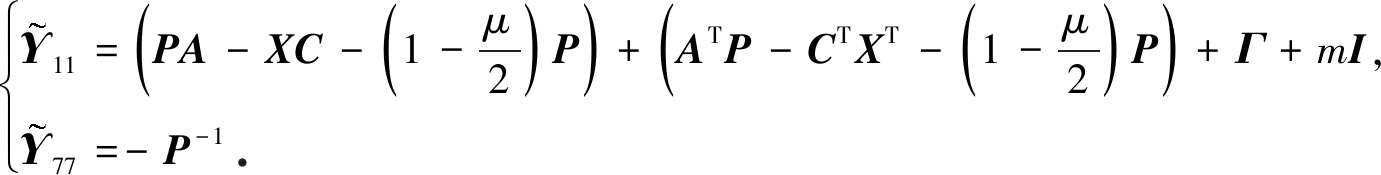(35)

## 3 仿 真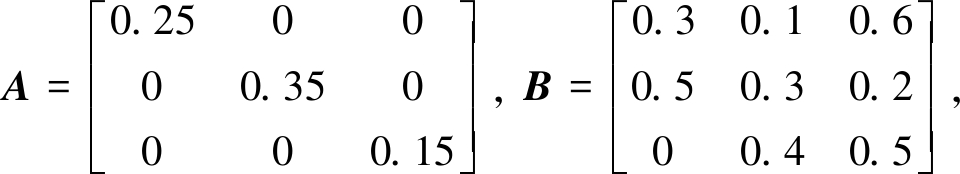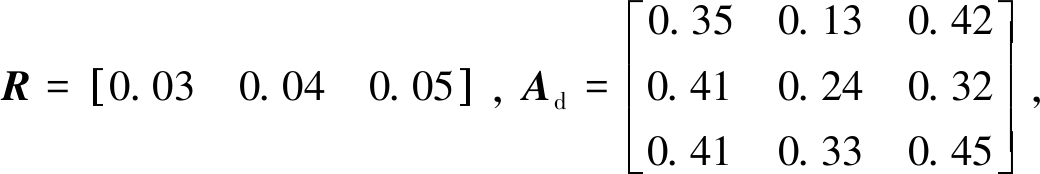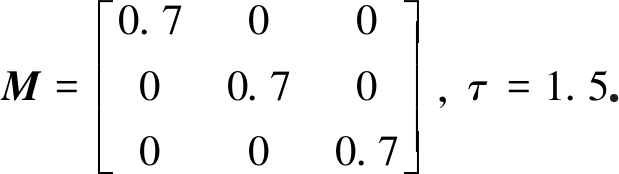ζ(x(s))=[tanh(x1(s)) tanh(x2(s)) tanh(x3(s))]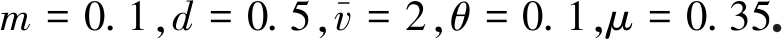测量输出式(2)的参数矩阵选择如下：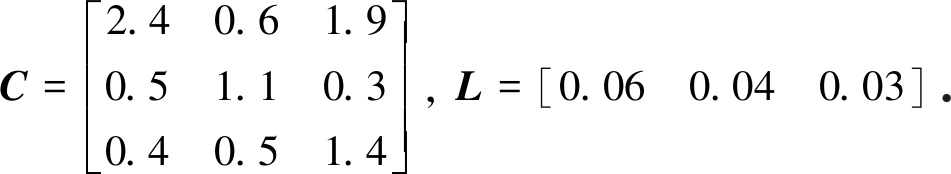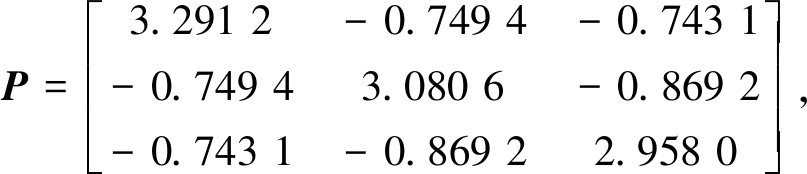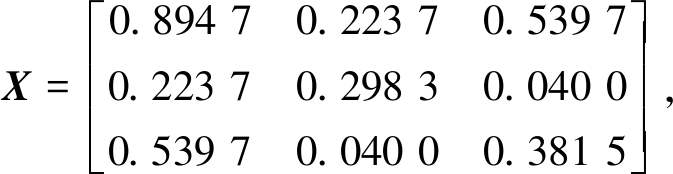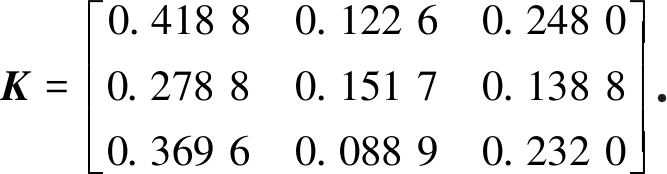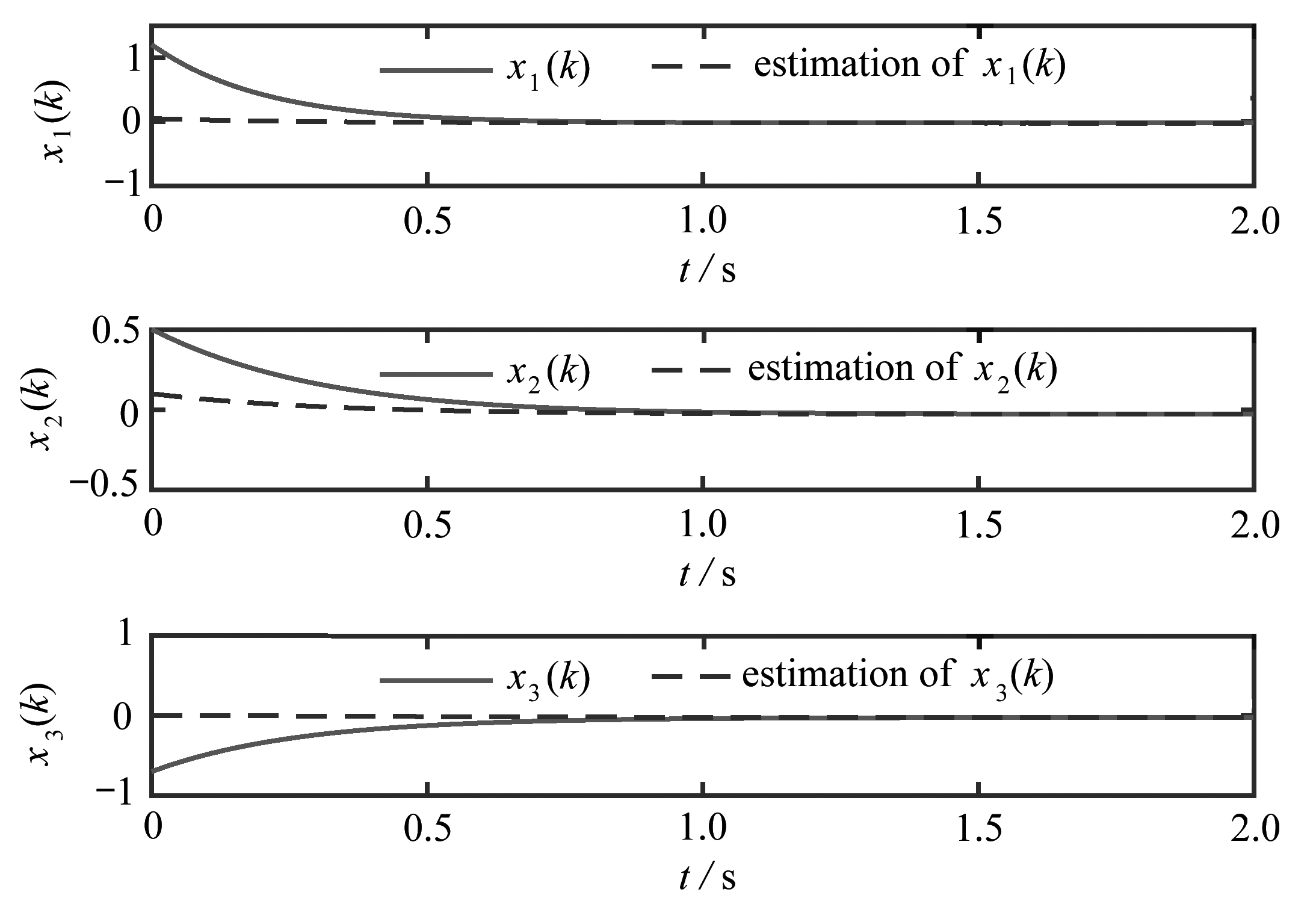Fig. 1 The system input and its state estimation curve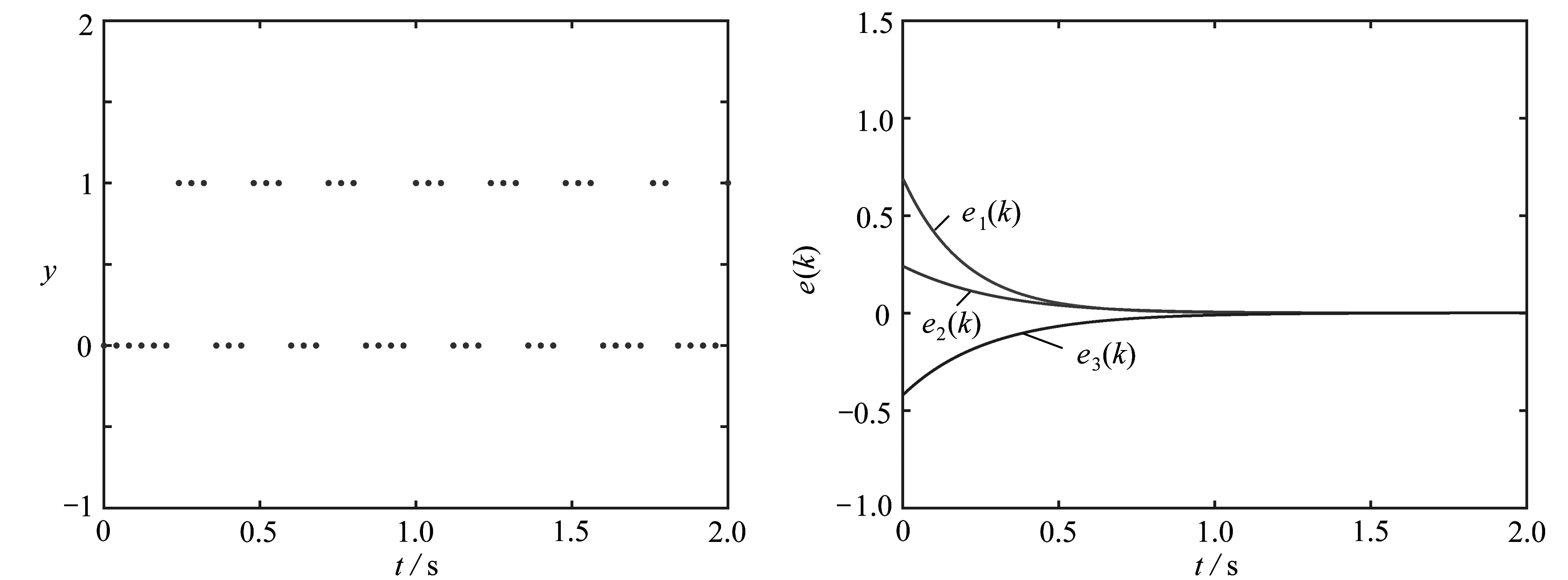Fig. 2 The event trigger mechanism Fig. 3 The system error curve

## 4 结 论

 IBRIR S, SU C Y. Simultaneous state and dead-zone parameter estimation for a class of bounded-state nonlinear systems[J]. IEEE Transactions on Control Systems Technology, 2011, 19(4): 911-919.

 ZHAO J B, ZHANG G X, DONG Z Y, et al. Forecasting-aided imperfect false data injection attacks against power system nonlinear state estimation[J]. IEEE Transactions on Smart Grid, 2016, 7(1): 6-8.

 PANKOV A, BOSOV A. Conditionally minimax algorithm for nonlinear system state estimation[J]. IEEE Transactions on Automatic Control, 1994, 39(8): 1617-1620.

 ZHENG W, WU W, GOMEZ-EXPOSITO A, et al. Distributed robust bilinear state estimation for power systems with nonlinear measurements[J]. IEEE Transactions on Power Systems, 2017, 32(1): 499-509.

 曾德强, 吴开腾, 宋乾坤, 等. 时滞神经网络随机抽样控制的状态估计[J]. 应用数学和力学, 2018, 39(7): 821-832.(ZENG Deqiang, WU Kaiteng, SONG Qiankun, et al. State estimation for delayed neural networks with stochastic sampled-data control[J]. Applied Mathematics and Mechanics, 2018, 39(7): 821-832.(in Chinese))

 LEUNG H, SHANMUGAM S, XIE N, et al. An ergodic approach for chaotic signal estimation at low SNR with application to ultra-wide-band communication[J]. IEEE Transactions on Signal Processing, 2006, 54(3): 1091-1103.

 BOLZERN P, COLANERI P, NICOLAO D G. On almost sure stability of continuous-time Markov jump linear systems[J]. Automatica, 2006, 42(6): 983-988.

 ZHAO X Y, DENG F Q. Moment stability of nonlinear discrete stochastic systems with time-delays based on H-representation technique[J]. Automatica, 2014, 50(2): 530-536.

 WANG Y Y, XIE L H, DE SOUZA C E. Robust control of a class of uncertain nonlinear systems[J]. Systems and Control Letters, 1992, 19(2): 139-149.

 WANG D, LIU D R, LI H L, et al. An approximate optimal control approach for robust stabilization of a class of discrete-time nonlinear systems with uncertainties[J]. IEEE Transactions on Systems, Man, and Cybernetics: Systems, 2015, 46(5): 713-717.

 KE M, ZHAO Y D, ZHAO X. Cooperation-driven distributed model predictive control for energy storage systems[J]. IEEE Transactions on Smart Grid, 2015, 6(6): 2583-2585.

 PUKDEBOON C, ZINOBER A S, THEIN M W L. Quasi-continuous higher order sliding-mode controllers for spacecraft-attitude-tracking maneuvers[J]. IEEE Transactions on Industrial Electronics, 2010, 57(4): 1436-1444.

 AMATO F, ARIOLA M, DORATO P. Finite-time control of linear systems subject to parametric uncertainties and disturbances[J]. Automatica, 2001, 37(9): 1459-1463.

 MAO Y, ZHANG H, ZHANG Z. Finite-time stabilization of discrete-time switched nonlinear systems without stable subsystems via optimal switching signal design[J]. IEEE Transactions on Fuzzy Systems, 2017, 25(1): 172-180.

 程桂芳, 慕小武. 一类不连续系统关于闭不变集的有限时间稳定性研究[J]. 应用数学和力学, 2009, 30(8): 1003-1008.(CHENG Guifang, MU Xiaowu. Finite-time stability with respect to a closed invariant set for a class of discontinuous systems[J]. Applied Mathematics and Mechanics, 2009, 30(8): 1003-1008.(in Chinese))

 WANG J, ZHANG H, WANG Z, et al. Finite-time synchronization of coupled hierarchical hybrid neural networks with time-varying delays[J]. IEEE Transactions on Cybernetics, 2017, 47(10): 2995-3004.

# Design of a Finite-Time State Estimator for Nonlinear Systems Under Event-Triggered Control

TONG Yinghao1, TONG Dongbing1, CHEN Qiaoyu2, ZHOU Wuneng3

(1. School of Electronic and Electrical Engineering, Shanghai University of Engineering Science, Shanghai 201620, P.R.China;2. School of Statistics and Mathematics, Shanghai Lixin University of Accounting and Finance, Shanghai 201620, P.R.China;3. School of Information Science and Technology, Donghua University,Shanghai 201620, P.R.China)

Abstract: The event-triggered state estimator for nonlinear systems with time delay was studied. Firstly, the state estimator for nonlinear systems was established by the event-triggered mechanism, and the Lyapunov function was used to make the system mean square bounded in finite time. Secondly, based on the H bounded condition, the system’s H finite time bounded criterion was obtained. Finally, a numerical example was given to illustrate the validity of the obtained result.

Key words: finite time; event-triggered mechanism; state estimator; H control; nonlinear system

*收稿日期： 2019-07-15； 修订日期：2020-05-10

DOI: 10.21656/1000-0887.400210

Foundation item: The National Natural Science Foundation of China(61673257;11501367); China Postdoctoral Science Foundation(2019M661322)